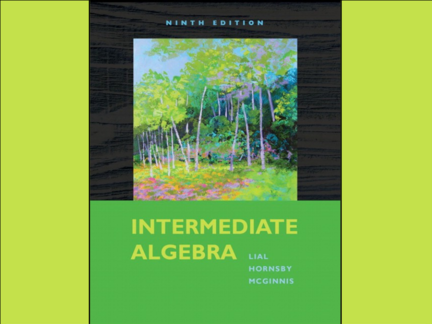# Exponential and Logarithmic Equations; Further ApplicationsContributed by:OBJECTIVES:
1. Solve equations involving variables in the exponents.
2. Solve equations involving logarithms.
3. Solve applications of compound interest.
4. Solve applications involving base e exponential growth and decay. 5. Use the change-of-base rule.
Sec 11.6 - 1
2. Chapter 11
Inverse, Exponential
and
Logarithmic Functions
Sec 11.6 - 2
3. 11.6
Exponential and Logarithmic
Equations; Further
Applications
Sec 11.6 - 3
4. 11.6 Exponential and Logarithmic Equations; Further
Applications
Objectives
1. Solve equations involving variables in the
exponents.
2. Solve equations involving logarithms.
3. Solve applications of compound interest.
4. Solve applications involving base e
exponential growth and decay.
5. Use the change-of-base rule.
5. 11.6 Exponential and Logarithmic Equations; Further
Applications
Solving Equations Involving Variables in the Exponents
6. 11.6 Exponential and Logarithmic Equations; Further
Applications
Solving an Exponential Equation
Begin by taking the
logarithm to the same
base on both sides.
7. 11.6 Exponential and Logarithmic Equations; Further
Applications
Solving an Exponential Equation (Base e)
Begin by taking the
logarithm to the same
base on both sides.
8. 11.6 Exponential and Logarithmic Equations; Further
Applications
Solving a Logarithmic Equation
9. 11.6 Exponential and Logarithmic Equations; Further
Applications
Solving a Logarithmic Equation
10. 11.6 Exponential and Logarithmic Equations; Further
Applications
Solving a Logarithmic Equation
11. 11.6 Exponential and Logarithmic Equations; Further
Applications
Solving a Logarithmic Equation
12. 11.6 Exponential and Logarithmic Equations; Further
Applications
Solving Equations Involving Logarithms
13. 11.6 Exponential and Logarithmic Equations; Further
Applications
Solving Equations Involving Logarithms
14. 11.6 Exponential and Logarithmic Equations; Further
Applications
Solving a Compound Interest Problem
15. 11.6 Exponential and Logarithmic Equations; Further
Applications
Solving a Compound Interest Problem for t
16. 11.6 Exponential and Logarithmic Equations; Further
Applications
Solving a Compound Interest Problem for t
17. 11.6 Exponential and Logarithmic Equations; Further
Applications
Solving a Compound Interest Problem for t
18. 11.6 Exponential and Logarithmic Equations; Further
Applications
Continuous Compounding
19. 11.6 Exponential and Logarithmic Equations; Further
Applications
Continuous Compounding
20. 11.6 Exponential and Logarithmic Equations; Further
Applications
Continuous Compounding
21. 11.6 Exponential and Logarithmic Equations; Further
Applications
The Change-of-Base Rule